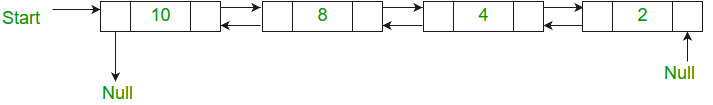# C++ Program For Merge Sort For Doubly Linked List

• Last Updated : 28 Dec, 2021

Given a doubly linked list, write a function to sort the doubly linked list in increasing order using merge sort.
For example, the following doubly linked list should be changed to 24810Merge sort for singly linked list is already discussed. The important change here is to modify the previous pointers also when merging two lists.

Below is the implementation of merge sort for doubly linked list.

## C++

 `// C++ program for merge sort on doubly  ` `// linked list  ` `#include ` `using` `namespace` `std; ` `class` `Node  ` `{  ` `    ``public``: ` `    ``int` `data;  ` `    ``Node *next, *prev;  ` `};  ` ` `  `Node *split(Node *head);  ` ` `  `// Function to merge two linked lists  ` `Node *merge(Node *first, Node *second)  ` `{  ` `    ``// If first linked list is empty  ` `    ``if` `(!first)  ` `        ``return` `second;  ` ` `  `    ``// If second linked list is empty  ` `    ``if` `(!second)  ` `        ``return` `first;  ` ` `  `    ``// Pick the smaller value  ` `    ``if` `(first->data < second->data)  ` `    ``{  ` `        ``first->next = merge(first->next, ` `                            ``second);  ` `        ``first->next->prev = first;  ` `        ``first->prev = NULL;  ` `        ``return` `first;  ` `    ``}  ` `    ``else` `    ``{  ` `        ``second->next = merge(first,second->next);  ` `        ``second->next->prev = second;  ` `        ``second->prev = NULL;  ` `        ``return` `second;  ` `    ``}  ` `}  ` ` `  `// Function to do merge sort  ` `Node *mergeSort(Node *head)  ` `{  ` `    ``if` `(!head || !head->next)  ` `        ``return` `head;  ` `    ``Node *second = split(head);  ` ` `  `    ``// Recur for left and right halves  ` `    ``head = mergeSort(head);  ` `    ``second = mergeSort(second);  ` ` `  `    ``// Merge the two sorted halves  ` `    ``return` `merge(head,second);  ` `}  ` ` `  `// A utility function to insert a new node  ` `// at the beginning of doubly linked list  ` `void` `insert(Node **head, ``int` `data)  ` `{  ` `    ``Node *temp = ``new` `Node(); ` `    ``temp->data = data;  ` `    ``temp->next = temp->prev = NULL;  ` `    ``if` `(!(*head))  ` `        ``(*head) = temp;  ` `    ``else` `    ``{  ` `        ``temp->next = *head;  ` `        ``(*head)->prev = temp;  ` `        ``(*head) = temp;  ` `    ``}  ` `}  ` ` `  `// A utility function to print a doubly  ` `// linked list in both forward and  ` `// backward directions  ` `void` `print(Node *head)  ` `{  ` `    ``Node *temp = head;  ` `    ``cout <<  ` `    ``"Forward Traversal using next pointer"``;  ` `    ``while` `(head)  ` `    ``{  ` `        ``cout << head->data << ``" "``;  ` `        ``temp = head;  ` `        ``head = head->next;  ` `    ``}  ` `    ``cout <<  ` `    ``"Backward Traversal using prev pointer"``;  ` `    ``while` `(temp)  ` `    ``{  ` `        ``cout << temp->data << ``" "``;  ` `        ``temp = temp->prev;  ` `    ``}  ` `}  ` ` `  `// Utility function to swap two integers  ` `void` `swap(``int` `*A, ``int` `*B)  ` `{  ` `    ``int` `temp = *A;  ` `    ``*A = *B;  ` `    ``*B = temp;  ` `}  ` ` `  `// Split a doubly linked list (DLL) into ` `// 2 DLLs of half sizes  ` `Node *split(Node *head)  ` `{  ` `    ``Node *fast = head,*slow = head;  ` `    ``while` `(fast->next && fast->next->next)  ` `    ``{  ` `        ``fast = fast->next->next;  ` `        ``slow = slow->next;  ` `    ``}  ` `    ``Node *temp = slow->next;  ` `    ``slow->next = NULL;  ` `    ``return` `temp;  ` `}  ` ` `  `// Driver program  ` `int` `main(``void``)  ` `{  ` `    ``Node *head = NULL;  ` `    ``insert(&head, 5);  ` `    ``insert(&head, 20);  ` `    ``insert(&head, 4);  ` `    ``insert(&head, 3);  ` `    ``insert(&head, 30);  ` `    ``insert(&head, 10);  ` `    ``head = mergeSort(head);  ` `    ``cout << ``"Linked List after sorting"``;  ` `    ``print(head);  ` `    ``return` `0;  ` `} ` `// This is code is contributed by rathbhupendra `

Output:

```Linked List after sorting
Forward Traversal using next pointer
3 4 5 10 20 30
Backward Traversal using prev pointer
30 20 10 5 4 3```

Time Complexity: Time complexity of the above implementation is same as time complexity of MergeSort for arrays. It takes Θ(nLogn) time.

Space Complexity:O(1). We are only using constant amount of extra space.
You may also like to see QuickSort for doubly linked list
Please refer complete article on Merge Sort for Doubly Linked List for more details!

My Personal Notes arrow_drop_up
Recommended Articles
Page :